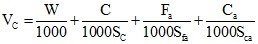# How to estimate construction quantities of materials for concreteAbsolute volume method is used to workout quantities of materials for generating necessary quantity of concrete based on specified mix ratios. This method is created on the basis of the percept that the volume of completely compacted concrete is identical to the absolute volume of all the materials of concrete, i.e. cement, sand, coarse aggregates and water.

A Concrete structure comprises of beams, slabs, columns and foundations etc. on the basis of type of structure. The volume of concrete necessary for concrete structure is estimated by totalling the volumes of every structural member or parts of members. The volume of a rectangular cross sectional member is measured by length x width x height (or depth or thickness). Appropriate formula is applied for various cross-sectional shapes of members.

The formula for calculation of materials for required volume of concrete is given by:Where, Vc denotes Absolute volume of fully compacted fresh concrete:
W denotes Mass of water
C denotes Mass of cement
Fa denotes Mass of fine aggregates
Ca denotes Mass of coarse aggregates
Sc, Sfa and Sca denote the particular gravities of cement, fine aggregates and coarse aggregates correspondingly.

This method of calculation for quantities of materials for concrete considers the mix proportions from design mix or nominal mixes for structural strength and need of strength.